# MQL5 中的电子表格

8 一月 2014, 09:39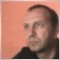0
1 048

### 1. 类的方法的说明

1.1. FirstResize

`void  FirstResize(const ENUM_DATATYPE &TYPE[]);`

1.2. SecondResize

`void  SecondResize(int j);`

1.3. FirstSize

```int   FirstSize();
```

1.4. SecondSize

`int   SecondSize();`

1.5. PruningTable

```void   PruningTable(int count);
```

1.6. CopyTable

`void   CopyTable(CTable *sor);`

`void   CopyTable(CTable *sor,int sec_beg,int sec_end);`

1.7. TypeTable

`ENUM_DATATYPE  TypeTable(int i)`

1.8. Change

Change() 方法执行列的交换。

`bool   Change(int &sor0,int &sor1);`

1.9. Insert

`bool   Insert(int rec,int sor);`

1.10. Variant/VariantCopy

```void   variant(int ind);
int    variant();
void   variantcopy(int rec,int sor);```

variant(int ind) 方法切换所选变体。执行自动内存分配。如果指定的参数少于上次指定的参数个数，将不会重新分配内存。

variantcopy 方法允许复制 "sor" 变体到 "rec" 变体。该函数被创建用于安排变体。如果 "rec" 变体不存在，它自动增加变体的数目，并且切换至新复制的变体。

1.11. SortTwoDimArray

SortTwoDimArray 方法按选定的行 "i" 排序表格。

`void   SortTwoDimArray(int i,int beg,int end,bool mode=false);`

1.12. QuickSearch

```int   QuickSearch(int i,long element,int beg,int end,bool mode=false);
```

1.13. SearchFirst

```int  SearchFirst(int i,long element,int beg,int end,bool mode=false);
```

1.14. SearchLast

```int   SearchLast(int i,long element,int beg,int end,bool mode=false);
```

1.15. SearchGreat

`int   SearchGreat(int i,long element,int beg,int end,bool mode=false);`

1.16. SearchLess

```int  SearchLess(int i,long element,int beg,int end,bool mode=false);
```

1.17. Set/Get

Set 和 Get 函数具有空类型；它们被表格使用的四种数据类型所覆盖。该函数识别数据类型，如果 "value" 参数不符合列类型，将显示一则警告消息且不会进行分配。唯一的例外是字符串类型。如果输入参数为字符串类型，它将被转换为列类型。该例外在不可能设置一个接收单元格的值的变量时，为使传输信息更加方便而量身定做。

```void   Set(int i,int j,long     value); // setting value of the i-th row and j-th column
void   Set(int i,int j,double   value); // setting value of the i-th row and j-th columns
void   Set(int i,int j,datetime value);// setting value of the i-th row and j-tj column
void   Set(int i,int j,string   value); // setting value of the i-th row and j-th column
```

```   //--- getting value
void   Get(int i,int j,long     &recipient); // getting value of the i-th row and j-th column
void   Get(int i,int j,double   &recipient); // getting value of the i-th row and j-th column
void   Get(int i,int j,datetime &recipient); // getting value of the i-th row and j-th column
void   Get(int i,int j,string   &recipient); // getting value of the i-th row and j-th column
```

1.19. sGet

`string sGet(int i,int j); // return value of the i-th row and j-th column `

Get 系列的唯一函数通过 "return" 运算符而不是参数变量返回值。返回一个字符串类型的值（不考虑列类型）。

1.20. StringDigits

```void  StringDigits(int i,int digits);
```

`int   StringDigits(int i);`

1.21. 使用示例：

```#include <Table.mqh>
ENUM_DATATYPE TYPE=
{TYPE_LONG,TYPE_LONG,TYPE_STRING,TYPE_DATETIME,TYPE_STRING,TYPE_STRING,TYPE_DOUBLE};
//     0          1          2            3              4            5            6   //7
void OnStart()
{
CTable table,table1;
table.FirstResize(TYPE);             // dividing table, determining column types
table.SecondResize(5);               // change the number of rows

table.Set(6,0,"321.012324568");        // assigning data to the 6-th column, 0 row
table.Insert(2,6);                   // insert 6-th column in the 2-nd position
table.PruningTable(3);               // cut the table to 3 columns
table.StringDigits(2,5);             // set precision of 5 digits after the decimal point
Print("table ",table.sGet(2,0));       // print the cell located in the 2-nd column, 0 row

table1.CopyTable(GetPointer(table));  // copy the entire table 'table' to the 'table1' table
table1.StringDigits(2,8);            // set 8-digit precision
Print("table1 ",table1.sGet(2,0));     // print the cell located in the 2-nd column, 0 row of the 'table1' table.
}```

```2011.02.09 14:18:37     Table Script (EURUSD,H1)        table1 321.01232000
2011.02.09 14:18:37     Table Script (EURUSD,H1)        table 321.01232```

### 2. 选择模型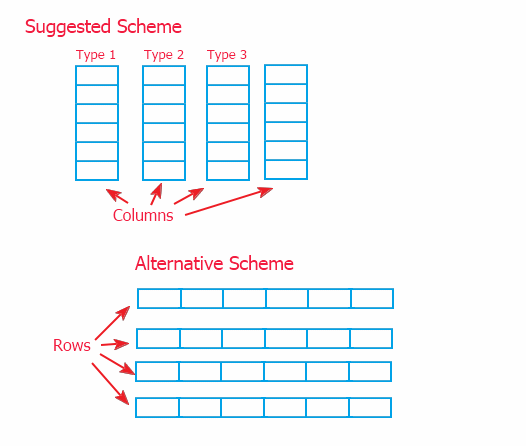### 3. 在动态数组中统一数据

```class CBase
{
public:
CBase(){Print(__FUNCTION__);};
~CBase(){Print(__FUNCTION__);};
virtual void       set(int sor){};
virtual void       set(double sor){};
virtual int        get(int k){return(0);};
virtual double     get(double k){return(0);};
};
//+------------------------------------------------------------------+
class CA: public CBase
{
private:
int              temp;
public:
CA(){Print(__FUNCTION__);};
~CA(){Print(__FUNCTION__);};
void              set(int sor){temp=sor;};
int               get(int k){return(temp);};
};
//+------------------------------------------------------------------+
class CB: public CBase
{
private:
double            temp;
public:
CB(){Print(__FUNCTION__);};
~CB(){Print(__FUNCTION__);};
void             set(double sor){temp=sor;};
double           get(double k){return(temp);};
};
//+------------------------------------------------------------------+
void OnStart()
{
CBase *a;   CBase *b;
a=new CA(); b=new CB();
a.set(15);  b.set(13.3);
Print("a=",a.get(0)," b=",b.get(0.));
delete a;
delete b;
}
```### 4. 操作数组

```before sorting by the 2-nd column
4 2 3
1 5 3
3 3 6
after sorting
1 5 3
3 3 6
4 2 3
Initial array looks as following:
a= 4; a= 2; a= 3;
a= 1; a= 5; a= 3;
a= 3; a= 3; a= 6;
And the array of indexes of sorting by the 2-nd column looks as:
r=1;
r=2;
r=0;
Sorted values are returned according to the following scheme:
a[r]-> 1; a[r]-> 5; a[r]-> 3;
a[r]-> 3; a[r]-> 3; a[r]-> 6;
a[r]-> 4; a[r]-> 2; a[r]-> 3;```

### 5. 排列二维数组

```class CarrayInt
{
public:
~CarrayInt(){};
int               array[];
};
//+------------------------------------------------------------------+
class CTwoarrayInt
{
public:
~CTwoarrayInt(){};
CarrayInt         array[];
};
//+------------------------------------------------------------------+
void OnStart()
{
CTwoarrayInt two;
two.array.array;
}```

### 6. 程序结构

CTable 类的代码使用（使用伪模板替代 C++ 模板）一文中介绍的模板编写。正是因为使用了模板，我才可以如此快速地编写这么一大段代码。这也是我不打算详细说明整段代码的原因；此外，算法的大部分代码都是对标准类的修改。CTable 类还执行数据处理方法（位于 CTable 类的实例中）和控制 Cint2D 类的索引的对象的协调。整个协调包装在覆盖公共方法中。

CTable 类中频繁重复的覆盖部分使用定义代替，以避免生成过于冗长的代码：

```#define _CHECK0_ Print(__FUNCTION__+"("+(string)i+","+(string)j+")");return;
#define _CHECK_ Print(__FUNCTION__+"("+(string)i+")");return(-1);
#define _FIRST_ first_data[aic[i]]
#define _PARAM0_ array_index.Ind(j),value
#define _PARAM1_ array_index.Ind(j),recipient
#define _PARAM2_ element,beg,end,array_index,mode```

`int QuickSearch(int i,long element,int beg,int end,bool mode=false){if(!check_type(i,TYPE_LONG)){_CHECK_}return(_FIRST_.QuickSearch(_PARAM2_));};`

`int QuickSearch(int i,long element,int beg,int end,bool mode=false){if(!check_type(i,TYPE_LONG)){Print(__FUNCTION__+"("+(string)i+")");return(-1);} return(first_data[aic[i]].QuickSearch(element,beg,end,array_index,mode));};`

array_index 对象存储第二个维度的元素的位置关系。第一个维度的索引编排由在 CTable 类的私有区段中声明的 aic[] 动态数组完成。它提供了改变列的位置的可能性（当然，不是实际移动，而是通过索引）。

`void CLONGArray::QuickSort(long &m_data[],Cint2D &index,int beg,int end,bool mode=0)`

```...
if(i<=j)
{
t=m_data[i];            it=index.Ind(i);
m_data[i++]=m_data[j];  index.Ind(i-1,index.Ind(j));
m_data[j]=t;            index.Ind(j,it);
if(j==0) break;
else     j--;
}
...```

### 总结

table.mqh (61.33 KB)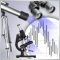小型趋势指标、中型趋势指标和主要趋势指标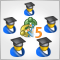基于交易模块创建多个 EA 交易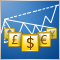在 MetaTrader 5 中实施多货币模式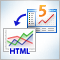HTML 中的图表